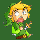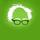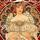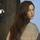## General Question# Why does Microsoft Excel sometimes calculate things differently?

Asked by ScottyMcGeester (1897) July 24th, 2015

I have 9 numbers in excel.
5.2
5.2
5.2
5.2
5.3
5.3
5.2
5.2
5.3

I wanted the average of them so excel did it. But the average came out 5.3. That didn’t sound right to me. There are way more 5.2s than 5.3s. So I used a calculator and the calculator told me 5.23 repeating, which I would round to 5.2. But when I click on the cell in excel and set it to add more significant figures, it gives me 5.26, hence why it rounds to 5.3.

The same kind of thing happens when I want to look up the %CV of certain things. It’s a long story but I basically took the absorbance of water and calculated the average and standard deviation. Both came out to .001.
%CV is the st. deviation divided by the average and multiply that by 100. But excel is telling me the %CV is 86.6 – which makes NO SENSE because when you divide .001 by .001 it obviously gets you 1 and 1 times 100 is 100.

What gives?

Observing members: 0Composing members: 0I can’t duplicate your problem. I added those nine numbers to excel, summed the column, and divided by 9. I get 5.233333 if I increase the decimal points, I keep getting more 3s. If I decrease to one decimal, I get 5.2. Seems right to me.

I also used Average() and got the same correct result. How are you calculating average in Excel?

DoNotKnow (3017)“Great Answer” (1) Flag as…I forgot to mention that there’s a 10th number but it’s an outlier – 5.5. But I removed it from the average calculation. So for example the formula reads: ”=average(I8:13, I15:I17)” I14 being the cell that the 5.5 is in.

Now this is weird. Because I just did this:

I opened up the file again and this time just wrote off the 9 numbers off to the side and did the average command again and THIS TIME it gives me 5.233 repeating. I wrote the formula as just ”=average(K1:K9)”

So going back to my original set of numbers in my chart, for some reason even though I explicitely asked it to skip the cell I14, it appears to still be integrating the 5.5.

I tried the same thing with my %CV issue. I wrote off .001 twice in two cells and divided them and then multiplied it by 100 and got 100. But then again, I simply wrote off the .001 while in original other chart the .001 was automatically calculated from my set of numbers. But there’s no reason why the caclulation should be off when everything is pointing to the right numbers in the formulas.

ScottyMcGeester (1897)“Great Answer” (1) Flag as…I did a couple of different things. I had ECELL average the numbers, came up with 5.233333333.

Then I averaged the numbers on the right side of the decimal point of that answer, =average(2,3,3,3,3,3,3,3,3) And came up with 2.8. So there is your 3. I think

I hope this makes sense!

Dutchess_III (45358)“Great Answer” (0) Flag as…@ScottyMcGeester I tried to reproduce the first result (the average of the nine numbers) in Quattro Pro, and the function returned 5.233, the expected result.

On the %CV question, the seemingly erroneous result does make sense because of the way software deals with decimal numbers. For all numbers, the software performs calculations using the entire number, not just the displayed portion. So if the actual standard deviation is .000866 for an average of .001, then dividing the standard deviation by the average and multiplying that result by 100 returns the result you received.

There is a straightforward way to see if this is the case, expand the width of the cells in question, then set the number of decimal places displayed to 10 or larger. That will reveal more of each number that Excel is working with.

sahID (2521)“Great Answer” (1) Flag as…or